Exponential equation

Solve exponential equation (in real numbers):

98x-2=9

Result

x =  0.38

Solution:Leave us a comment of example and its solution (i.e. if it is still somewhat unclear...):Be the first to comment!To solve this verbal math problem are needed these knowledge from mathematics:

Do you have a linear equation or system of equations and looking for its solution? Or do you have quadratic equation?

Next similar examples:

1. Exponential equationIn the set R solve the equation: ?
2. Coordinate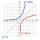Determine missing coordinate of the point M [x, 120] of the graph of the function f bv rule: y = 5x
3. Insert into GPBetween numbers 5 and 640 insert as many numbers to form geometric progression so sum of the numbers you entered will be 630. How many numbers you must insert?
4. Water lilies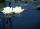Water lilies are growing on the pond and their number is doubled every day. The whole layer is covered in 12 days. How many days will it cover 8 layers?
5. Powers 3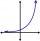2 to the power of n divided by 4 to the power of -3 equal 4. What is the vaule of n?
6. The city 3The city has 22,000 residents. How long it is expected to have 25,000 residents if the average annual population growth is 1.4%?
7. Logif ?, what is b?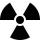After 548 hours decreases the activity of a radioactive substance to 1/9 of the initial value. What is the half-life of the substance?
9. LogarithmDetermine the number whose decimal logarithm is -3.8.
10. Logarithmic equation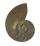Solve equation: log33(3x + 21) = 0
11. Solve 3Solve quadratic equation: (6n+1) (4n-1) = 3n2
12. Linear imaginary equationGiven that ? "this is z star" Find the value of the complex number z.
13. Intercept with axisF(x)=log(x+4)-2, what is the x intercept
14. AsymptoteWhat is the vertical asymptote of ?
15. Ball gameRichard, Denis and Denise together scored 932 goals. Denis scored 4 goals over Denise but Denis scored 24 goals less than Richard. Determine the number of goals for each player.
16. Is complexAre these numbers 2i, 4i, 2i + 1, 8i, 2i + 3, 4 + 7i, 8i, 8i + 4, 5i, 6i, 3i complex?
17. Theorem proveWe want to prove the sentence: If the natural number n is divisible by six, then n is divisible by three. From what assumption we started?